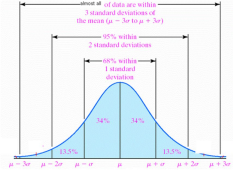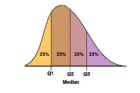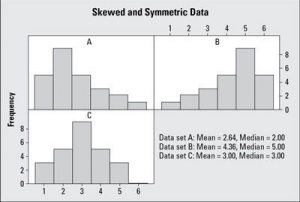×

×

# 1.5xiqr rule Description

Stat. 2000 Study Guide: Test 1

## what is Experimental Study?Most Important Concepts

● What a Z­Score​ is and how to calculate it.

● How to use the 1.5xIQR Rule​ to identify outliers

● Understand what a residual​ is and its significance

● Identify all types of studies

● Understanding of all vocabulary

Calculations

● Finding the Interquartile Range:​ Subtract the third quartile (Q3) from the first quartile (Q1) ○ 50% of all values within the data set fall within the IQR.

● Identifying Outliers:​ Any values that are less than Q1­1.5(IQR)​ or greater than Q3+1.5(IQR)​ are considered outliers

○ This is called the 1.5x IQR Rule

● Finding the Quartiles## what is Cluster Sampling?Don't forget about the age old question of arruto

○ σ​ represents the standard deviation

○ μ​ represents the mean.

● Z­Score:

○ The Z score is used to calculate how many standard deviations a value falls from the mean.

○ Positive scores are above the mean and negative scores are below the mean. ● Determining the Strength of a Correlation Coefficient:​ The correlation coefficient, or r, is interpreted using the equation  ​ ­1<​ r <1 Don't forget about the age old question of static budget

○ Values closer to ­1 show a strong negative correlation

○ Values closer to 1 show a strong positive correlation

○ If r=0, then the variables have no correlation.

Vocabulary

Experimental Study​: Researchers manipulate variables within the study in order to gather their data. This method ensures that no outside factors can be used to explain the results obtained, and is used to determine cause and effect relationships between variables

## what is Sampling Bias?Observational Study​: Researchers observe data that the subjects exhibit naturally. The researcher cannot manipulate the subject or variables in any way, and this method cannot determine cause and effect relationships

Simple Random Sampling: ​A sampling method in which each member of the population has an equal chance of being selected to participate in the study.

Stratified Sampling​: A sampling method in which researchers divide the population into groups based on common characteristics such as height or age. Then, subjects are randomly selected within each group to participate in the study.

Cluster Sampling​: A method of sampling in which the population is divided into random groups. Then, a group is randomly selected and all members of this group participate in the study.

Systematic Sampling​: A method of sampling in which subjects are selected from the population using an unbiased rule. For example, researchers would select every 10th member of the population to participate. If you want to learn more check out swk ir

Convenience Sampling​: A flawed method of sampling in which the subjects are individuals that are the easiest to contact. We also discuss several other topics like uncg cst 105

Undercoverage:​ This refers to group(s) in the population that are not represented in the study

Sampling Bias:​ This bias is caused by selecting subjects from the population in a method that isn’t random or when undercoverage is present. If you want to learn more check out ­ Central Nervous System­ nervous system that includes the brain and spinal cord After brain, what develops?

Nonresponse Bias:​ A bias that occurs when subjects either cannot be reached or refuse to participate in the survey.

Response Bias​: A bias that occurs when participation in a survey is voluntary, so members of the population that respond may have strong opinions about the survey topic that are not present in the majority of the population.

Statistics​: The science of learning from data.

Design​: The method in which the research data is gathered

Description​: A summary of the data obtained from the study and any correlations that have been discovered.

Inference​: Using data that has been previously obtained to make predictions or decisions.

Population​: Every member of the group the researcher is interested in studying

Sample​: The amount of the population in which researchers obtained data. This is made up of the subjects.

Subject:​ A member of the population that participates in the study. These individuals make up the sample.

Parameter:​ A characteristic or value that a researcher would like to obtain from the population, such as the average income of every person living in Georgia. This is usually impossible to obtain. Don't forget about the age old question of utep cs

Statistic​: A numerical value that summarizes the data researchers have collected from the subject. Experimental Unit​: The individual whom is studied by the survey.

Treatment​: A condition applied to the subject.

Explanatory Variable​: A variable in the study that explains the changes a researcher has observed. Response Variable​: The variable in the study which data is collected from by researchers.

Placebo​: A “dummy” treatment given to the control group so they aren’t aware which treatment they are receiving.

Double Blind​: A research method in which neither the researchers or the patients know which group is the control group or the experimental group.

Single Blind​: A research method in which the researchers know which group is the experimental or control, but the patients don’t.

Confounding​: A condition that occurs when multiple explanations can be given for the results gathered by the study. This prevents researchers from determining a cause and effect relationship between variables.

Random Design​: Subjects in a study are randomly assigned to either the placebo group or a treatment group.

Matched­Pairs Design​: Subjects participate in the experiment in pairs. These pairs were already related in some way before the start of the study, such as a husband and wife.

Blocking​: Researchers separate the subjects into homogeneous groups and the study is performed on both groups separately. For example, the researchers could create a male group and a female group.

Variable​: A characteristic or observation that is studied

Categorical Variable​: A variable that has a finite set of responses, such as a yes or no question.

Quantitative Variable​: A variable with a numerical value that cannot be put into a finite number of groups, such as a student’s SAT score.

Skewed​: A graph that is not equally distributed on both sides.

Symmetrical​: A graph whose values are equally distributed.

Mode​: The tallest bar on a graph and is also referred to as a peak.

Mean​: The average of all values in a data set

Median​: The value that falls in the exact middle of the data set.

Range​: The difference between the largest value and the smallest value in a data set.

Standard Deviation​: A calculation that represents how far the observations fall from the mean. First Quartile​: The quartile that contains 25% of all data gathered

Second Quartile​: The quartile that contains 50% of the data

Third Quartile​: The quartile that contains 75% of the data.

Interquartile Range​: A calculation of the distance between the first and third quartiles. This is calculated by the equation Q3­Q1

Outliers​: Observed values that fall beyond the normal range of the data. They can be identified using the 1.5(IQR) method.

Z Score​: A calculation of how many standard deviations from the mean a value lies. It is calculated by subtracting the observed value from the mean and dividing by the standard deviation.

Positive Association:​ If the value of the explanatory variable increases, the value of the response variable increases as well.

Negative Association​: When the value of the explanatory value increases, the response variable decreases

No Association​: The response variable and the explanatory variable show no relation.

Correlation Coefficient:​ A numerical value that is used to determine the strength of a relationship between two variables and is evaluated using the equation ­1< r <1

Residual​: The difference between the actual value and the predicted value. ● A positive residual means that the predicted value was too small

● A negative residual means that the predicted value was too large.

Interpreting Graphs

A: Using Position to Determine Variability

● Percentile:​ Means that x percent of all observations fall at or below that value ○ For example, if you score in the 90th percentile, it means that 90% of people ● Data is separated into three groups called quartiles.​ They split the data set into four parts, with each part containing 25% of the observed values.

○ First Quartile (Q1):​ 25th percentile of the data

○ Second Quartile (Q2):​ 50th percentile of the data. This falls at the median ○ Third Quartile (Q3) :​ 75th percentile of the data.● Interquartile Range (IQR):​ The distance from Q1 to Q3.

○ 50% of all data falls in this range, so this is a useful way of generalizing a data set. ○ Calculated by the equation IQR= Q3­Q1

● Outliers:​ Observations that are either much larger or smaller than the majority of values that are observed

○ Outliers are detected by using the 1.5xIQR method

■ Observations that are less than Q1­1.5(IQR)​ or greater than Q3+1.5(IQR)​ are classified as outliers.

B: Using Shape to Determine Variability

Looking at the overall shape of the histogram allows us to observe the trends found in the data and interpret the meaning of the study.

● Skewed​: not symmetric, meaning the graph is not equal on both sides. Skewed histograms are either right skewed or left skewed.

● Symmetrical​: The graph shows equal distribution across the values

● Mode​: The tallest bar in the graph, also known as a peak. Histograms can contain multiple peaks

○ If the graph has one peak, it is called unimodal

○ Two peaks are bimodal​, three peaks are trimodal, etc.

● Tails​: The collection of bars on either side of the mode

○ Symmetrical graphs have tails of equal length

○ Right skewed graphs have a longer tail on the right side

○ Left skewed graphs have longer tails on the left sideThe above histograms are described as:

● A​: Unimodal and right skewed

● B​: Unimodal and left skewed

● C​: Unimodal and symmetrical

Page ExpiredIt looks like your free minutes have expired! Lucky for you we have all the content you need, just sign up here
References: